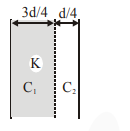# For changing the capacitance of a given parallel plate capacitor,Question:

For changing the capacitance of a given parallel plate capacitor, a dielectric material of dielectric constant K is used, which has the same area as the plates of the capacitor. The thickness of the

dielectric slab is $\frac{3}{4} \mathrm{~d}$, where ' $\mathrm{d}$ ' is the separation

between the plates of parallel plate capacitor. The new capacitance (C') in terms of original capacitance (C0 ) is given by the following relation :

1. $\mathrm{C}^{\prime}=\frac{3+\mathrm{K}}{4 \mathrm{~K}} \mathrm{C}_{0}$

2. $\mathrm{C}^{\prime}=\frac{4+\mathrm{K}}{3} \mathrm{C}_{0}$

3. $\mathrm{C}^{\prime}=\frac{4 \mathrm{~K}}{\mathrm{~K}+3} \mathrm{C}_{0}$

4. $C^{\prime}=\frac{4}{3+K} C_{0}$

Correct Option: 3,

Solution:$C_{0}=\frac{\epsilon_{0} A}{d}$

$\mathrm{C}^{\prime}=\mathrm{C}_{1}$ and $\mathrm{C}_{2}$ in series.

i.e. $\frac{1}{C^{\prime}}=\frac{1}{C_{1}}+\frac{1}{C_{2}}$

$\frac{1}{\mathrm{C}^{\prime}}=\frac{(3 \mathrm{~d} / 4)}{\epsilon_{0} \mathrm{KA}}+\frac{\mathrm{d} / 4}{\epsilon_{0} \mathrm{~A}}$

$\frac{1}{C^{\prime}}=\frac{d}{4 \in_{0} A}\left(\frac{3+K}{K}\right)$

$C^{\prime}=\frac{4 K C_{0}}{(3+K)}$